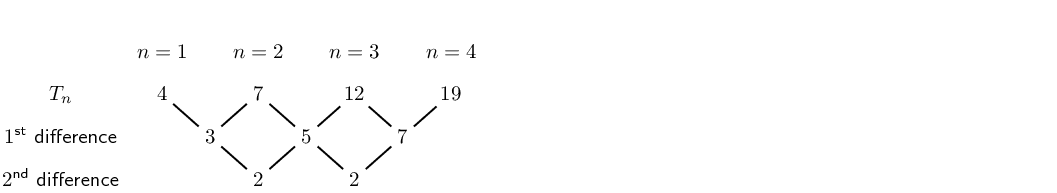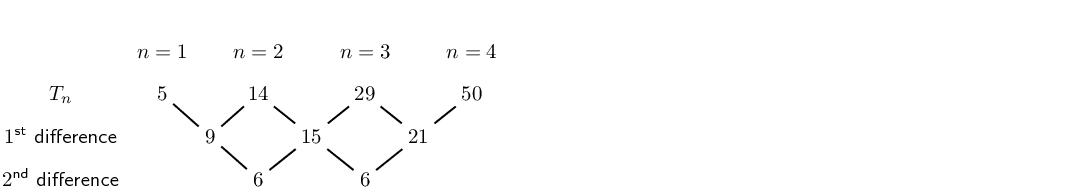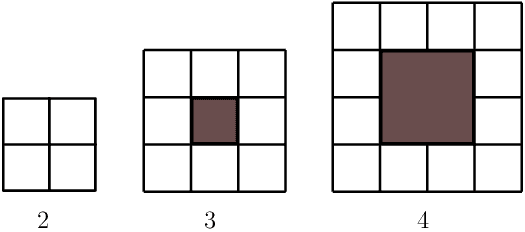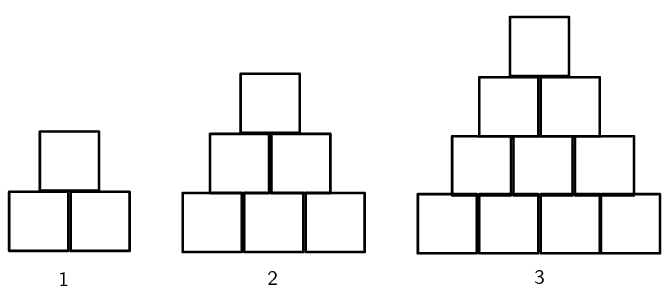Home Practice
For learners and parents For teachers and schools
Textbooks
Full catalogue
Pricing SupportLog in

We think you are located in United States. Is this correct?

# End of chapter exercises

## End of chapter exercises

Textbook Exercise 3.4

Find the first five terms of the quadratic sequence defined by:

$${T}_{n}={n}^{2}+2n+1$$
$$-4; 9; 16; 25; 36$$

Determine whether each of the following sequences is:

• a linear sequence,
• or neither.

$$6;9;14;21;30;...$$

\begin{align*} \text{First differences: } &= 3; 5; 7; 9; \\ \text{Second difference: } &= 2 \end{align*}

$$1;7;17;31;49;...$$

\begin{align*} \text{First differences: } &= 6; 10; 14; 18 \\ \text{Second difference: } &= 4 \end{align*}

$$8;17;32;53;80;...$$

\begin{align*} \text{First differences: } &= 9; 15; 21; 27 \\ \text{Second difference: } &= 6 \end{align*}

$$9;26;51;84;125;...$$

\begin{align*} \text{First differences: } &= 17; 25; 33; 41 \\ \text{Second difference: } &= 8 \end{align*}

$$2;20;50;92;146;...$$

\begin{align*} \text{First differences: } &= 18; 30; 42; 54 \\ \text{Second difference: } &= 12 \end{align*}

$$5;19;41;71;109;...$$

\begin{align*} \text{First differences: } &= 14; 22; 30; 38 \\ \text{Second difference: } &= 8 \end{align*}

$$2;6;10;14;18;...$$

$\text{First difference: } = 4$

Linear sequence

$$3;9;15;21;27;...$$

$\text{First difference: } = 6$

Linear sequence

$$1;\text{2,5};5;\text{8,5};13;...$$

\begin{align*} \text{First differences: } &= \text{1,5};~ \text{2,5};~ \text{3,5};~ \text{4,5} \\ \text{Second difference: } &= 1 \end{align*}

$$10;24;44;70;102;...$$

\begin{align*} \text{First differences: } &= 14; 20; 26; 32 \\ \text{Second difference: } &= 16 \end{align*}

$$2\frac{1}{2}; 6; 10\frac{1}{2}; 16; 22\frac{1}{2}; \ldots$$

\begin{align*} \text{First differences: } &= \text{3,5};~ \text{4,5};~ \text{5,5};~ \text{6,5} \\ \text{Second difference: } &= 1 \end{align*}

$$3p^2; 6p^2; 9p^2; 12p^2; 15p^2; \ldots$$

$\text{First difference: } = 3p^2$

Linear sequence

$$2k; 8k; 18k; 32k; 50k; \ldots$$

\begin{align*} \text{First differences: } &= 6k; 10k; 14k; 18k \\ \text{Second difference: } &= 4k \end{align*}

Given the pattern: $$16; x; 46; \ldots$$, determine the value of $$x$$ if the pattern is linear.

\begin{align*} x - 16 &= 46 - x \\ 2x &= 62 \\ \therefore x &= 31 \end{align*}

Given $$T_n = 2n^2$$, for which value of $$n$$ does $$T_n = 242$$?

\begin{align*} 2n^2 &= 242 \\ n^2 &= 121 \\ \therefore n &= 11 \end{align*}

Given $${T}_{n}=3{n}^{2}$$, find $${T}_{11}$$.

\begin{align*} T_n &= 3n^2 \\ \therefore T_11 &= 3(11)^2 \\ &= 363 \end{align*}

Given $$T_n = n^2+4$$, for which value of $$n$$ does $$T_n = 85$$?

\begin{align*} n^2 + 4 &= 85 \\ n^2 &= 81 \\ \therefore n &= 9 \end{align*}

Given $${T}_{n}=4{n}^{2}+3n-1$$, find $${T}_{5}$$.

\begin{align*} T_n &= 4n^2 + 3n - 1 \\ \therefore T_5 &= 4(5)^2 + 3(5) - 1\\ &= 100 + 15 - 1 \\ &= 114 \end{align*}

Given $$T_n = \dfrac{3}{2}n^2$$, for which value of $$n$$ does $$T_n = 96$$?

\begin{align*} \dfrac{3}{2}n^2 &= 96 \\ n^2 &= 96 \times \frac{2}{3} \\ &= 64 \\ \therefore n &= 8 \end{align*}

For each of the following patterns, determine:

• the next term in the pattern,
• and the general term,
• the tenth term in the pattern.

$$3; 7; 11; 15; \ldots$$

\begin{align*} d &= 7 - 3 \\ &= 4 \\ T_5 &= 15 + 4 \\ &= 19 \\ T_n &= 4n - 1 \\ T_{10} &= 4(10) - 1 \\ &= 39 \end{align*}

$$17; 12; 7; 2; \ldots$$

\begin{align*} d &= 12 - 17 \\ &= -5 \\ T_5 &= 2 - 5 \\ &= -3 \\ T_n &= 22 - 5n \\ T_{10} &= 22 - 5(10) \\ &= -28 \end{align*}

$$\frac{1}{2}; 1; 1\frac{1}{2}; 2; \ldots$$

\begin{align*} d &= 1 - \frac{1}{2} \\ &= \frac{1}{2} \\ T_5 &= 2 + \frac{1}{2} \\ &= 2 \frac{1}{2} \\ T_n &= \frac{1}{2}n \\ T_{10} &= \frac{1}{2}(10) \\ &= 5 \end{align*}

$$a; a+b; a+2b; a+3b; \ldots$$

\begin{align*} d &= a + b - a \\ &= b \\ T_5 &= a + 3b + b \\ &= a + 4b \\ T_n &= a - b + bn \\ T_{10} &= a - b + b(10) \\ &= a + 9b \end{align*}

$$1; -1; -3; -5; \ldots$$

\begin{align*} d &= -1 - 1 \\ &= -2 \\ T_5 &= -5 -2 \\ &= -7 \\ T_n &= 3 - 2n \\ T_{10} &= 3 - 2(10) \\ &= -17 \end{align*}

For each of the following sequences, find the equation for the general term and then use the equation to find $${T}_{100}$$.

$$4;7;12;19;28;...$$$$2;8;14;20;26;...$$

\begin{align*} d &= 8 - 2 \\ &= 6 \\ \therefore T_n &= 6n - 4 \end{align*} \begin{align*} T_n &= 6n - 4 \\ \therefore T_{100} &= 6(100) - 4 \\ &= 596 \end{align*}

$$7;13;23;37;55;...$$$$5;14;29;50;77;...$$Given: $$T_n = 3n-1$$

Write down the first five terms of the sequence.

$$2; 5; 8; 11; 14$$

What do you notice about the difference between any two consecutive terms?

Constant difference, $$d=3$$

Will this always be the case for a linear sequence?

Yes

Given the following sequence: $$-15; -11; -7; \ldots ; 173$$

Determine the equation for the general term.

\begin{align*} d &= -11 - (-15) \\ &= 4 \\ T_n &= 4n - 19 \end{align*}

Calculate how many terms there are in the sequence.

\begin{align*} T_n &= 4n - 19 \\ 173 &= 4n - 19 \\ 192 &= 4n \\ \therefore 48 &= n \end{align*}

Given $$3; 7; 13; 21; 31; \ldots$$

Thabang determines that the general term is $$T_n = 4n - 1$$. Is he correct? Explain.

\begin{align*} \text{First differences: } &= 4; 6; 8; 10 \\ \text{Second difference: } &= 2 \end{align*}

Thabang's general term $$T_n = 4n - 1$$ describes a linear sequence and this is a quadratic sequence.

Cristina determines that the general term is $$T_n = n^2 + n + 1$$. Is she correct? Explain.

\begin{align*} T_n &= an^2 + bn + c \\ \text{Second difference: } 2a &= 2 \\ \therefore a &= 1 \\ 3a + b &= 4 \\ b &= 4 - 3(1) \\ \therefore b &= 1 \\ a + b + c &= 3 \\ \therefore c &= 3 - a - b \\ &= 3 - 1 - 1 \\ \therefore c &= 1 \\ \therefore T_n &= n^2 + n + 1 \end{align*}

Given the following pattern of blocks:Draw pattern $$\text{5}$$.Complete the table below:

 pattern number $$(n)$$ $$\text{2}$$ $$\text{3}$$ $$\text{4}$$ $$\text{5}$$ $$\text{10}$$ $$\text{250}$$ $$n$$ number of white blocks $$(w)$$ $$\text{4}$$ $$\text{8}$$
 pattern number $$(n)$$ $$\text{2}$$ $$\text{3}$$ $$\text{4}$$ $$\text{5}$$ $$\text{10}$$ $$\text{250}$$ $$n$$ number of white blocks $$(w)$$ $$\text{4}$$ $$\text{8}$$ $$\text{12}$$ $$\text{16}$$ $$\text{36}$$ $$\text{996}$$ $$4n - 4$$

Is this a linear or a quadratic sequence?

Linear

Cubes of volume $$\text{1}$$ $$\text{cm^{3}}$$ are stacked on top of each other to form a tower:Complete the table for the height of the tower:

 tower number $$(n)$$ $$\text{1}$$ $$\text{2}$$ $$\text{3}$$ $$\text{4}$$ $$\text{10}$$ $$n$$ height of tower $$(h)$$ $$\text{2}$$
 tower number $$(n)$$ $$\text{1}$$ $$\text{2}$$ $$\text{3}$$ $$\text{4}$$ $$\text{10}$$ $$n$$ height of tower $$(h)$$ $$\text{2}$$ $$\text{3}$$ $$\text{4}$$ $$\text{5}$$ $$\text{11}$$ $$n+1$$

What type of sequence is this?

Linear

Now consider the number of cubes in each tower and complete the table below:

 tower number $$(n)$$ $$\text{1}$$ $$\text{2}$$ $$\text{3}$$ $$\text{4}$$ number of cubes $$(c)$$ $$\text{3}$$
 tower number $$(n)$$ $$\text{1}$$ $$\text{2}$$ $$\text{3}$$ $$\text{4}$$ number of cubes $$(c)$$ $$\text{3}$$ $$\text{6}$$ $$\text{10}$$ $$\text{15}$$

What type of sequence is this?

Determine the general term for this sequence.

\begin{align*} T_n &= an^2 + bn + c \\ \text{Second difference: } 2a &= 1 \\ \therefore a &= \frac{1}{2} \\ 3a + b &= 3 \\ b &= 3 - 3(\frac{1}{2}) \\ \therefore b &= \frac{3}{2} \\ a + b + c &= 3 \\ \therefore c &= 3 - a - b \\ &= 3 - \frac{1}{2} - \frac{3}{2} \\ \therefore c &= 1 \\ \therefore T_n &= \frac{1}{2}n^2 + \frac{3}{2}n + 1 \end{align*}

How many cubes are needed for tower number $$\text{21}$$?

\begin{align*} T_n &= \frac{1}{2}n^2 + \frac{3}{2}n + 1 \\ \therefore T_{21} &= \frac{1}{2}(21)^{2}+ \frac{3}{2}(21) + 1 \\ &= \frac{441}{2} + \frac{63}{2} + \frac{2}{2} \\ &= 253 \end{align*}

How high will a tower of $$\text{496}$$ cubes be?

\begin{align*} T_n &= \frac{1}{2}n^2 + \frac{3}{2}n + 1\\ 496 &= \frac{1}{2}n^2 + \frac{3}{2}n + 1 \\ 0 &= \frac{1}{2}n^2 + \frac{3}{2}n - 495 \\ &= n^2 + 3n - 990 \\ &= (n - 30)(n + 33) \\ \therefore n = 30 &\text{ or } n = -33 \\ \therefore n &= 30 \\ \text{And } h &= n + 1 \\ &= 30 + 1 \\ &= \text{31}\text{ cm} \end{align*}

A quadratic sequence has a second term equal to $$\text{1}$$, a third term equal to $$-\text{6}$$ and a fourth term equal to $$-\text{14}$$.

Determine the second difference for this sequence.

\begin{align*} T_3 - T_2 &= -6 -(1) \\ &= -7 \\ T_4 - T_3 &= -14 - (-6) \\ &= -8 \\ \therefore \text{Second difference} &= -1 \end{align*}

Hence, or otherwise, calculate the first term of the pattern.

\begin{align*} T_{1} &= 1 + 6 \\ &= 7 \end{align*}

There are $$\text{15}$$ schools competing in the U16 girls hockey championship and every team must play two matches — one home match and one away match.

Use the given information to complete the table:

 no. of schools no. of matches $$\text{1}$$ $$\text{0}$$ $$\text{2}$$ $$\text{3}$$ $$\text{4}$$ $$\text{5}$$
 no. of schools no. of matches $$\text{1}$$ $$\text{0}$$ $$\text{2}$$ $$\text{2}$$ $$\text{3}$$ $$\text{6}$$ $$\text{4}$$ $$\text{12}$$ $$\text{5}$$ $$\text{20}$$

Calculate the second difference.

\begin{align*} T_2 - T_1 &= 2 - 0 \\ &= 2 \\ T_3 - T_2 &= 6 - 2 \\ &= 4 \\ T_4 - T_3 &= 12 -6 \\ &= 6 \\ T_5 - T_4 &= 20 - 12 \\ &= 8 \\ \therefore \text{Second difference} &= 2 \end{align*}

Determine a general term for the sequence.

\begin{align*} T_n &= an^2 + bn + c \\ \text{Second difference: } 2a &= 2 \\ \therefore a &= 1 \\ 3a + b &= 2 \\ b &= 2 - 3(1) \\ \therefore b &= -1 \\ a + b + c &= 0 \\ \therefore c &= - a - b \\ &= -1 - (-1) \\ \therefore c &= 0 \\ \therefore T_n &= n^2 -n \end{align*}

How many matches will be played if there are $$\text{15}$$ schools competing in the championship?

\begin{align*} T_n &= n^2 -n \\ T_{15} &= (15)^2 - 15 \\ &= 225 - 15 \\ &= 210 \end{align*}

If $$\text{600}$$ matches must be played, how many schools are competing in the championship?

\begin{align*} T_n &= n^2 -n \\ 600 &= n^2 -n \\ 0 &= n^2 -n - 600\\ &= (n - 25)(n + 24)\\ \therefore n = 25 &\text{ or } n = -24 \\ \therefore n &= 25 \end{align*}

The first term of a quadratic sequence is $$\text{4}$$, the third term is $$\text{34}$$ and the common second difference is $$\text{10}$$. Determine the first six terms in the sequence.

\begin{align*} \text{Let } T_2 &= x \\ \therefore T_2 - T_1 &= x - 4 \\ \text{And } T_3 - T_2 &= 34 - x \\ \text{Second difference } &= (T_3 - T_2) - (T_2 - T_1) \\ &= (34 - x) - (x - 4)\\ \therefore 10 &= 38 - 2x \\ 2x &= 28 \\ \therefore x &= 14 \end{align*}

$$4; 14; 34; 64; 104; 154$$

Challenge question:

Given that the general term for a quadratic sequences is $$T_n = an^2 + bn + c$$, let $$d$$ be the first difference and $$D$$ be the second common difference.

Show that $$a = \dfrac{D}{2}$$.

\begin{align*} T_n &= an^2 + bn +c \\ T_1 &= a(1)^2 + b(1) +c \\ &= a + b + c \\ T_2 &= a(2)^2 + b(2) +c \\ &= 4a + 2b + c\\ T_3 &= a(3)^2 + b(3) +c \\ &= 9a + 6b + c\\ \text{First difference } d &= T_2 - T_1 \\ \therefore d &= (4a + 2b + c) - (a + b + c ) \\ &= 3a + b \\ \therefore b &= d - 3a \\ \text{Second difference } D &= (T_3 - T_2) - (T_2 - T_1)\\ \therefore D &= (5a + b) - (3a + b) \\ &= 2a \\ \therefore a &= \dfrac{D}{2} \end{align*}

Show that $$b = d - \dfrac{3}{2}D$$.

\begin{align*} a &= \dfrac{D}{2} \\ b &= d - 3a \\ \therefore b &= d - 3 \left( \dfrac{D}{2} \right) \\ &= d - \dfrac{3}{2} D \end{align*}

Show that $$c = T_1 - d + D$$.

\begin{align*} T_1 &= a + b + c \\ \therefore c &= T_1 - a - b \\ &= T_1 - \left( \dfrac{D}{2} \right) - \left( d - \dfrac{3}{2}D \right) \\ &= T_1 - \dfrac{D}{2} - d + \dfrac{3}{2}D \\ &= T_1 - d + D \end{align*}

Hence, show that $$T_n = \dfrac{D}{2}n^2 + \left(d - \dfrac{3}{2}D\right)n + \left(T_1 -d + D\right)$$.

\begin{align*} T_n &= an^2 + bn +c \\ \therefore T_n &= \dfrac{D}{2}n^2 + \left( d - \dfrac{3}{2}D \right)n + \left( T_1 - d + D \right) \end{align*}